AP State Syllabus AP Board 6th Class Maths Solutions Chapter 8 Basic Geometric Concepts Ex 8.4 Textbook Questions and Answers.

## AP State Syllabus 6th Class Maths Solutions 8th Lesson Basic Geometric Concepts Ex 8.4Question 1.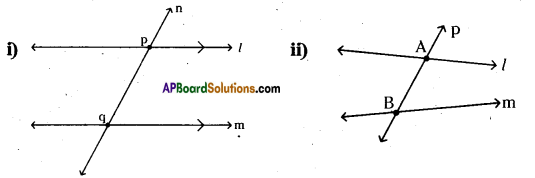Measure all the angles in the above figures.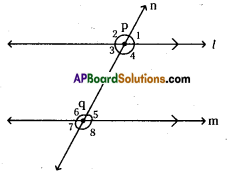∠1 = 70°
∠3 = 70°
∠5 = 70°
∠7 = 70°

∠2 = 110°
∠4 = 110°
∠6 = 110°
∠8= 110°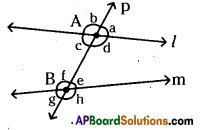∠a = 60°
∠c = 60°
∠e = 50°
∠g = 50°

∠b = 120°
∠d = 120°
∠f = 130°
∠h = 130°Question 2.
Sum of which two angles is 180° in each figure ?
Solution:
From above question,
i) ∠1 + ∠2 = 180°

i) ∠1 + ∠2 = 180°
∠2 + ∠3 = 180°
∠1 + ∠4 = 180°
∠3 + ∠4 = 180°

∠5 + ∠6 = 180°
∠6 + ∠7 = 180°
∠7 + ∠8 = 180°
∠5 + ∠8 = 180°

∠1 + ∠8 = 180°
∠4 + ∠5 = 180°
∠2 + ∠7 = 180°
∠3 + ∠6 = 180°

ii) ∠a + ∠b = 180°
∠b + ∠c = 180°
∠c + ∠d = 180°
∠a + ∠d = 180°

∠e + ∠f = 180°
∠f + ∠g = 180°
∠g + ∠h = 180°
∠e + ∠h = 180°Question 3.
In the given figure measure ∠FOG and draw the same in your note book.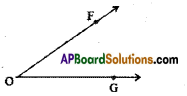Solution: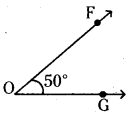Question 4.
In the given figure measure the angles ∠AOB, ∠BOC.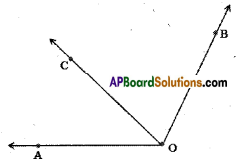Solution: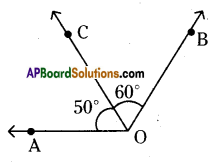∠AOB = 110°
∠BOC = 60°
∠AOC = 50°Question 5.
Write some acute, obtuse and reflexive angles atleast 2 for each.
Solution:
Acute angles : 10°, 30°, 45°, 60°, 89° (< 90°)
Obtuse angles : 110°, 150°, 160°, 172°, 178° (90° < obtuse < 180°) Reflex angles : 210°, 270°, 300°, 345°, 359° (reflex > 180°)